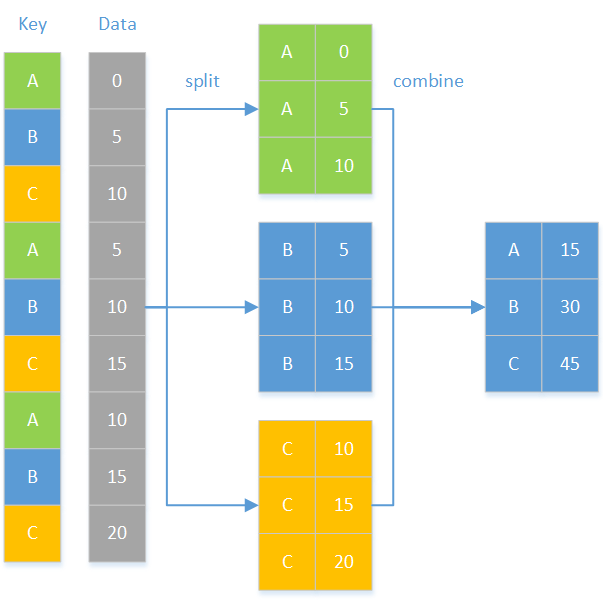### Python 分组处理

groupby 是 pandas 中非常重要的一个函数, 主要用于数据分类和聚合计算. 其思想是“split-apply-combine”（拆分 – 应用 – 合并），如下图：EID NAME SURNAME GENDER STATE BIRTHDAY HIREDATE DEPT SALARY 1 Rebecca Moore F California 1974/11/20 2005/3/11 R&D 7000 2 Ashley Wilson F New York 1980/7/19 2008/3/16 Finance 11000 3 Rachel Johnson F New   Mexico 1970/12/17 2010/12/1 Sales 9000 4 Emily Smith F Texas 1985/3/7 2006/8/15 HR 7000 5 Ashley Smith F Texas 1975/5/13 2004/7/30 R&D 16000 … … … … … … … … …

Python代码

 import pandas as   pd employee =   pd.read_csv(“Employees.csv”) dept_emp_num =   employee.groupby(‘DEPT’)[‘DEPT’].count() print(dept_emp_num) 读取数据 分组计数

Python 代码

 import pandas as pd employee = pd.read_csv(“Employees.csv”) dept_gender_salary =   employee.groupby([‘DEPT’,’GENDER’],as_index=False).SALARY.mean() print(dept_gender_salary) 多列分组再聚合

Python 代码

 import pandas as pd import numpy as np employee = pd.read_csv(“Employees.csv”) employee[‘BIRTHDAY’]=pd.to_datetime(employee[‘BIRTHDAY’]) years_salary =   employee.groupby(np.floor((employee[‘BIRTHDAY’].dt.year-1900)/10)).SALARY.mean() print(years_salary) 生日列转换成日期格式   计算衍生数组并按此数组分组，再计算平均工资

（一）   多列单聚合

Python 代码

 import pandas as pd employee = pd.read_csv(“Employees.csv”) dept_agg =   employee.groupby(‘DEPT’,as_index=False).agg({‘EID’:’count’,’SALARY’:’mean’}) print(dept_agg.rename(columns={‘EID’:’NUM’,’SALARY’:’AVG_SALARY’})) 分组并对 EID 计数，对 SALARY 求平均 重命名列名

（二）   单列多聚合

Python 代码

 import pandas as   pd employee =   pd.read_csv(“Employees.csv”) dept_agg = employee.groupby(‘DEPT’).SALARY.agg([‘count’,’mean’]).reset_index() print(dept_agg.rename(columns={‘count’:’NUM’,’mean’:’AVG_SALARY’})) 对 SALARY 计数并求平均 重命名列名

（三）   多列多聚合

Python 代码

 import pandas as   pd import datetime def max_age(s):     today = datetime. datetime.today().year     age = today-s.dt.year     return age.max() employee = pd.read_csv(“Employees.csv”) employee[‘BIRTHDAY’]=pd.to_datetime(employee[‘BIRTHDAY’]) dept_agg =   employee.groupby(‘DEPT’).agg({‘SALARY’:[‘count’,’mean’],’BIRTHDAY’:max_age})       dept_agg.columns   = [‘NUM’,’AVG_SALARY’,’MAX_AGE’] print(dept_agg.reset_index()) 函数：求最大年龄 年份 求年龄       按 DEPT 分组，根据 SALARY 计数和求均值，BIRTHDAY 使用 max_age 计算最大年龄 修改列名

Python 代码

 import pandas as pd employee = pd.read_csv(“Employees.csv”) employee[‘AVG_SALARY’] =   employee.groupby(‘DEPT’).SALARY.transform(‘mean’) print(employee) 按照 DEPT 分组并对 SALARY 求平均

Python 代码

 import pandas as pd employee = pd.read_csv(“Employees.csv”) employee[‘HIREDATE’]=pd.to_datetime(employee[‘HIREDATE’]) employee_new =   employee.groupby(‘DEPT’,as_index=False).apply(lambda   x:x.sort_values(‘HIREDATE’)).reset_index(drop=True) print(employee_new) 修改入职时间格式   按 DEPT 分组，并对各组按照 HIREDATE 排序，最后重置索引

Python 代码

 import pandas as pd def salary_diff(g):     max_age =   g[‘BIRTHDAY’].idxmin()     min_age =   g[‘BIRTHDAY’].idxmax()     diff =   g.loc[max_age][‘SALARY’]-g.loc[min_age][‘SALARY’]     return diff employee = pd.read_csv(“Employees.csv”) employee[‘BIRTHDAY’]=pd.to_datetime(employee[‘BIRTHDAY’]) salary_diff = employee.groupby(‘DEPT’).apply(salary_diff) print(salary_diff) 函数：计算各组工资差 年龄最大的索引 年龄最小的索引 计算工资差       按 DEPT 分组并使用自定义函数计算

source: https://stackoverflow.com/questions/59110612/pandas-groupby-mode-every-n-rows

time                       a                       b

0                          0.5                    -2.0

1                          0.5                    -2.0

2                          0.1                    -1.0

3                          0.1                    -1.0

4                          0.1                    -1.0

5                          0.5                    -1.0

6                          0.5                    -1.0

7                          0.5                    -3.0

8                          0.5                    -1.0

time                       a                       b

2                          0.5                    -2.0

5                          0.1                    -1.0

8                          0.5                    -1.0

Python 代码

 import pandas as pd import numpy as np data = pd.read_csv(“group3.txt”,sep=’\t’) res = data.groupby(np.arange(len(data)) //   3).agg(lambda x: x.mode().iloc[-1]) print(res) 按照衍生列分组，使用 agg 结合 lambda 的方式得到众数，取各组各列的最后 1 个众数作为结果

duration  location  user

0        10    house    A

1         5    house    A

2         5      gym    A

3         4      gym    B

4        10     shop    B

5         4      gym    B

6         6      gym    B

duration  location   user

15    house    A

5      gym    A

4      gym    B

10     shop    B

10      gym    B

 import pandas as pd df = pd.DataFrame({‘user’ : [‘A’, ‘A’, ‘A’, ‘B’, ‘B’,   ‘B’,’B’],                 ‘location’ : [‘house’,’house’,’gym’,’gym’,’shop’,’gym’,’gym’],                 ‘duration’:[10,5,5,4,10,4,6]}) derive = (df.location !=   df.location.shift()).cumsum() res = df.groupby([‘user’, ‘location’, derive],   as_index=False, sort=False)[‘duration’].sum() print(res) 生成数据       创造衍生列 按照 user,location 和衍生列分组，对 duraton 求和

source: https://stackoverflow.com/questions/62461647/choose-random-rows-in-pandas-datafram

ID          code

333_c_132   x

333_c_132   n06

333_c_132   n36

333_c_132   n60

333_c_132   n72

333_c_132   n84

333_c_132   n96

333_c_132   n108

333_c_132   n120

999_c_133   x

999_c_133   n06

999_c_133   n12

999_c_133   n24

998_c_134   x

998_c_134   n06

998_c_134   n12

998_c_134   n18

998_c_134   n36

997_c_135   x

997_c_135   n06

997_c_135   n12

997_c_135   n24

997_c_135   n36

996_c_136   x

996_c_136   n06

996_c_136   n12

996_c_136   n18

996_c_136   n24

996_c_136   n36

995_c_137   x

333_c_132   n06

999_c_133   n12

998_c_134   n18

997_c_135   n36

996_c_136   n18

 import pandas as pd df = pd.read_csv(“data.txt”) derive = df.code.eq(‘x’).cumsum() res=df[df.code.ne(‘x’)].groupby(derive).apply(lambda   x : x.sample(1)) res=res.reset_index(level=0, drop=True) print(res) 生成衍生列 根据衍生列分组，使用 apply 结合 lambda 的方式随机抽样 重置索引

1）没有空子集

2）原集合的任何成员都属于且只属于某一个子集

1）可能出现空子集（比如基准集合的某些成员在待分组集合中并不存在）；

2）可能有待分组集合成员未被分到任何子集（比如有些不重要的成员未被列入基准集合）；

3）每个成员最多只出现在一个子集中。

（一）出现空子集

Python 代码

 import pandas as pd def align_group(g,l,by):     d = pd.DataFrame(l,columns=[by])     m =   pd.merge(d,g,on=by,how=’left’) return m.groupby(by,sort=False) employee = pd.read_csv(“Employees.csv”) l = [‘M’,’F’] res = employee.groupby(‘DEPT’).apply(lambda   x:align_group(x, l, ‘GENDER’).apply(lambda s:s.EID.count())) print(res) 函数，对位分组 生成对照的 dataframe 利用 merge 完成对位运算 分组   指定序列 按 DEPT 分组，再对各组使用函数对位分组，对 EID 进行计数

（二）有待分组集合成员未被分到任何子集

 import pandas as pd def align_group(g,l,by):     d =   pd.DataFrame(l,columns=[by])     m =   pd.merge(d,g,on=by,how=’left’)     return   m.groupby(by,sort=False) employee = pd.read_csv(“Employees.csv”) sub_dept = [‘Administration’, ‘HR’, ‘Marketing’,   ‘Sales’] res =   align_group(employee,sub_dept,’DEPT’).apply(lambda x:x.EID.count()) print(res) 函数，对位分组         指定顺序的部门子集 使用对位分组函数分组，再对 EID 计数

 import pandas as pd import datetime def eval_g(dd:dict,ss:str):     return   eval(ss,dd)    emp_file = ‘E:\\txt\\employee.txt’ emp_info = pd.read_csv(emp_file,sep=’\t’) employed_list = [‘Within five years’,’Five to ten   years’,’More than ten years’,’Over fifteen years’] employed_str_list =   [“(s<5)”,”(s>=5) &   (s<10)”,”(s>=10)”,”(s>=15)”] today = datetime.datetime.today().year arr = pd.to_datetime(emp_info[‘HIREDATE’]) employed = today-arr.dt.year emp_info[‘EMPLOYED’]=employed dd = {‘s’:emp_info[‘EMPLOYED’]} group_cond = [] for n in range(len(employed_str_list)):     emp_g =   emp_info.groupby(eval_g(dd,employed_str_list[n]))     emp_g_index   = [index for index in emp_g.size().index]     if True not   in emp_g_index:           female_emp=0           male_emp=0     else:         group =   emp_g.get_group(True)         sum_emp   = len(group)           female_emp = len(group[group[‘GENDER’]==’F’])           male_emp = sum_emp-female_emp       group_cond.append([employed_list[n],male_emp,female_emp]) group_df =   pd.DataFrame(group_cond,columns=[‘EMPLOYED’,’MALE’,’FEMALE’]) print(group_df) 函数，字符串转表达式           分组条件       计算入职时间       循环分组条件 按分组条件分组 分组索引 如果没有满足条件的成员 男女员工数为 0   满足条件 获取分组 计算男女员工人数         汇总各个分组条件的计算结果

Python 在进行分组处理时，多数情况可以比较优雅的处理，但在处理有序分组时，如值变化分组、条件变化分组时则需要自己想办法生成满足分组条件的衍生列，略显麻烦。对位分组和枚举分组的两种情况更是糟糕，需要自己想办法去绕，要么使用 merge 运算，要么多次分组，使分组的成本变得很高，这样看来，Pandas 的分组运算还有其局限性。

 问题 SPL代码 简单说明 一 A.groups(DEPT;count(~):NUM) A是数据表，按 DEPT 分组，count() 计数 二 A.groups(DEPT,GENDER;avg(SALARY):AVG_SALARY) 按 DEPT,GENDER 分组，avg() 平均 三 A.groups((year(BIRTHDAY)- 1900)\10:years;avg(SALARY):AVG_SALARY) (year(BIRTHDAY)-1900)\10命名为 years，并分组 四 A.groups(DEPT;count(EID):NUM,avg(SALARY):AVG_SAL ARY) 多列单聚合 A.groups(DEPT;count(SALARY):NUM,avg(SALARY):AVG_ SALARY) 单列多聚合 A.groups(DEPT;count(SALARY):NUM,avg(SALARY):AVG_ SALARY,max(age(BIRTHDAY)):MAX_AVG) 多列多聚合 五 B=A.derive(AVG_SALARY) >B.group(DEPT).((a=~.avg(SALARY),~.run(AVG_SALARY =a))) 增加新列 修改为分组的聚合值 六 A.group(DEPT).conj(~.sort(HIREDATE)) 分组排序 A.group(DEPT;(ma=~.minp(BIRTHDAY),mi=~.maxp(BIR THDAY),ma.SALARY-mi.SALARY):SALARY_DIF) 分组子集运算

 问题 SPL代码 简单说明 七 A.groups@n((#-1)\3;y) 每三行分 1 组，y 是聚合运算表达式 八 A.groups@o(user,location;y) @o选项：值变化分组 九 A.group@i(code==”x”).(y) @i选项：条件变化分组

 问题 SPL分组处理 简单说明 十 s=[“M”,”F”] A.group(DEPT).(~.align@a(s,GENDER).(y)) 可能出现空子集 s=[“Administration”,   “HR”, “Marketing”, “Sales”] A.align@a(s,DEPT).(y) 有待分组集合成员未被分到任何子集 十一 c=[“?<5”,”?>=5   && ?<10”,”?>=10”,”?>=15”] A.enum(c, EMPLOYED) 有成员被分到不同子集

Python 如何处理大文件